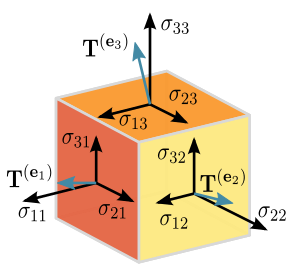# Tensor

## What is a Tensor?

A Tensor is a mathematical object similar to, but more general than, a vector and often represented by an array of components that describe functions relevant to coordinates of a space. Put simply, a Tensor is an array of numbers that transform according to certain rules under a change of coordinates. A Tensor can be defined as a single point, a collection of isolated points, or it may be defined as a continuum of points in which elements of the tensor are functions of position, and the Tensor forms what is known as a Tensor field. This means that rather than being represented by a single point, the tensor is defined at multiple points together in a space.Source

### Orders of Tensors

Tensors come in varying forms and levels of complexity defined by their related order. For example, a Tensor of order zero, often represented as a single number, is called a scalar. In short, a scalar is the value of an object as a function of a position, because scalars continuously vary from point-to-point within the scalar field. A Tensor of order one is known as a vector, often defined in a three-dimensional space with three numbers/functions of position. A vector consists of magnitude (length) and direction, meaning that it cannot be represented by a single number like a scalar. A Tensor of order two is called a matrix. Matrices are used to represent characteristics of an object that often is affected by multiple factors or forces. For example, the forces upon a gyroscope are the direction of spin (a vector) and an applied force or torque.

### Tensors and Machine Learning

As a cornerstone of machine learning system libraries like Tensorflow, Tensors are used by neural networks as components to describe and calculate phenomena. For example, a machine learning algorithm might use Tensors of order two, also called vectors, in calculating the descriptive characteristics of an electric field. As any point in the field is defined by both magnitude and direction, a vector, rather than a scalar, is the appropriate form of Tensor to be applied.